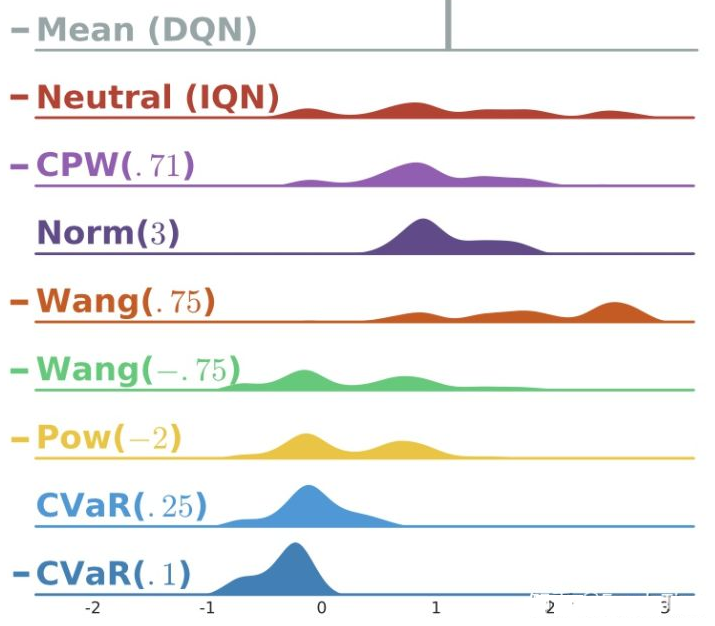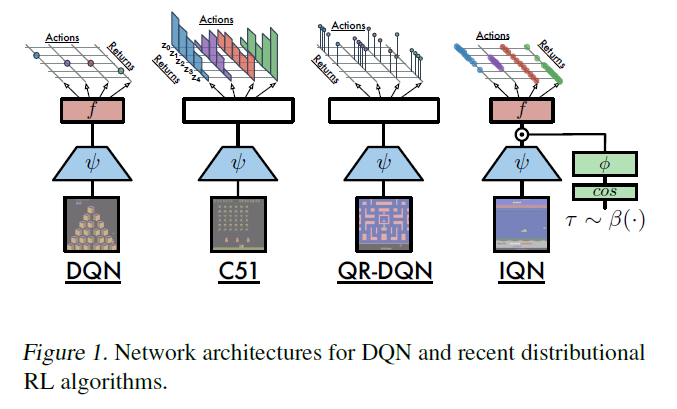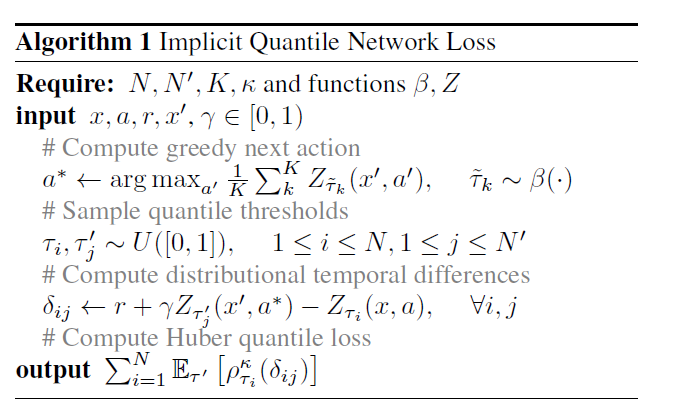# 强化学习的学习之路（二十五）_2021-01-25：Distributional DQN：Implicit Quantile Networks for Distributional RL

• 版本一
如果有两个随机变量 X , Y X, Y , 我们相比 Y Y 更偏好 X X , 写作 X ≻ Y X \succ Y , 那么这代表对任何随 机变量 Z , X Z, X Z Z 的混合都优于 Y Y Z Z 的混合, 这种 “优于" 表示为
α F X + ( 1 − α ) F Z ≥ α F Y + ( 1 − α ) F Z , ∀ α ∈ [ 0 , 1 ] \alpha F_{X}+(1-\alpha) F_{Z} \geq \alpha F_{Y}+(1-\alpha) F_{Z}, \forall \alpha \in[0,1]
在这种情况下，我们可以找到一个效用函数 U U 来描述这种偏好，那么策略可以表示为
π ( x ) = arg ⁡ max ⁡ a E Z ( x , a ) [ U ( z ) ] \pi(x)=\underset{a}{\arg \max } \underset{Z(x, a)}{\mathbb{E}}[U(z)]

• 版本二

如果有两个随机变量 X , Y X, Y , 我们相比 Y Y 更偏好 X X , 写作 X ≻ Y X \succ Y , 那么这代表对任何随 机变量 Z , X Z, X Z Z 的混合都优于 Y Y Z Z 的混合, 这种 “优于" 表示为
α F X − 1 + ( 1 − α ) F Z − 1 ≥ α F Y − 1 + ( 1 − α ) F Z − 1 , ∀ α ∈ [ 0 , 1 ] \alpha F_{X}^{-1}+(1-\alpha) F_{Z}^{-1} \geq \alpha F_{Y}^{-1}+(1-\alpha) F_{Z}^{-1}, \forall \alpha \in[0,1]
在这种情况下，我们可以找到一个distortion risk measure h h 来描述这种偏好，那么策略可以表示为
π ( x ) = arg ⁡ max ⁡ a ∫ − ∞ ∞ z ∂ ∂ z ( h ∘ F Z ( x , a ) ) ( z ) d z \pi(x)=\underset{a}{\arg \max } \int_{-\infty}^{\infty} z \frac{\partial}{\partial z}\left(h \circ F_{Z(x, a)}\right)(z) d z

π ( x ) = arg ⁡ max ⁡ a E Z ( x , a ) [ z ] = arg ⁡ max ⁡ a Q ( x , a ) \pi(x)=\underset{a}{\arg \max } \underset{Z(x, a)}{\mathbb{E}}[z]=\underset{a}{\arg \max } Q(x, a)

π ( x ) = arg ⁡ max ⁡ a ∫ − ∞ ∞ z ∂ ∂ z F Z ( x , a ) ( z ) d z = arg ⁡ max ⁡ a ∫ − ∞ ∞ z P Z ( x , a ) ( z ) d z = arg ⁡ max ⁡ a E Z ( x , a ) [ z ] = arg ⁡ max ⁡ a Q ( x , a ) \begin{aligned} \pi(x) &=\underset{a}{\arg \max } \int_{-\infty}^{\infty} z \frac{\partial}{\partial z} F_{Z(x, a)}(z) d z \\ &=\underset{a}{\arg \max } \int_{-\infty}^{\infty} z P_{Z(x, a)}(z) d z \\ &=\underset{a}{\arg \max } \underset{Z(x, a)}{\mathbb{E}}[z] \\ &=\underset{a}{\arg \max } Q(x, a) \end{aligned}

令  z = F Z − 1 ( τ ) ∫ 0 1 F Z − 1 ( τ ) d β ( τ ) = z = F Z − 1 ( τ ) ∫ − ∞ ∞ z d β ( F Z ( z ) ) = ∫ − ∞ ∞ z ∂ ∂ z ( β ∘ F Z ) ( z ) d z \begin{array}{l} \text { 令 } z=F_{Z}^{-1}(\tau) \\ \int_{0}^{1} F_{Z}^{-1}(\tau) d \beta(\tau) \stackrel{z=F_{Z}^{-1}(\tau)}{=} \int_{-\infty}^{\infty} z d \beta\left(F_{Z}(z)\right) \\ =\int_{-\infty}^{\infty} z \frac{\partial}{\partial z}\left(\beta \circ F_{Z}\right)(z) d z \end{array}

Q β ( x , a ) : = E τ ∼ U ( [ 0 , 1 ] ) [ Z β ( τ ) ( x , a ) Q_{\beta}(x, a):=\underset{\tau \sim U([0,1])}{\mathbb{E}}\left[Z_{\beta(\tau)}(x, a)\right.

Q β ( x , a ) : = E τ ∼ U ( [ 0 , 1 ] ) [ Z β ( τ ) ( x , a ) ] = ∫ 0 1 F Z − 1 ( τ ) d β ( τ ) Q_{\beta}(x, a):=\underset{\tau \sim U([0,1])}{\mathbb{E}}\left[Z_{\beta(\tau)}(x, a)\right]=\int_{0}^{1} F_{Z}^{-1}(\tau) d \beta(\tau)

π β ( x ) = arg ⁡ max ⁡ a ∈ A ∫ − ∞ ∞ z ∂ ∂ z ( β ∘ F Z ) ( z ) d z = arg ⁡ max ⁡ a ∈ A ∫ 0 1 F Z − 1 ( τ ) d β ( τ ) = arg ⁡ max ⁡ a ∈ A τ ∼ U ( [ 0 , 1 ] ) [ Z β ( τ ) ( x , a ) ] = arg ⁡ max ⁡ a ∈ A Q β ( x , a ) \begin{aligned} \pi_{\beta}(x) &=\underset{a \in \mathcal{A}}{\arg \max } \int_{-\infty}^{\infty} z \frac{\partial}{\partial z}\left(\beta \circ F_{Z}\right)(z) d z \\ &=\underset{a \in \mathcal{A}}{\arg \max } \int_{0}^{1} F_{Z}^{-1}(\tau) d \beta(\tau) \\ &=\underset{a \in \mathcal{A}}{\arg \max }_{\tau \sim U([0,1])}\left[Z_{\beta(\tau)}(x, a)\right] \\ &=\underset{a \in \mathcal{A}}{\arg \max } Q_{\beta}(x, a) \end{aligned}

• CPW函数：
CPW ⁡ ( η , τ ) = τ η ( τ η + ( 1 − τ ) η ) 1 η \operatorname{CPW}(\eta, \tau)=\frac{\tau^{\eta}}{\left(\tau^{\eta}+(1-\tau)^{\eta}\right)^{\frac{1}{\eta}}}

• Wang函数：

(其中 Φ \Phi 是标准正态分布的CDF函数)
Wang ⁡ ( η , τ ) = Φ ( Φ − 1 ( τ ) + η ) \operatorname{Wang}(\eta, \tau)=\Phi\left(\Phi^{-1}(\tau)+\eta\right)

• Pow函数：
Pow ⁡ ( η , τ ) = { τ 1 1 + ∣ η ∣ ,  if  η ≥ 0 1 − ( 1 − τ ) 1 1 + ∣ η ∣ ,  otherwise  \operatorname{Pow}(\eta, \tau)=\left\{\begin{array}{ll}\tau^{\frac{1}{1+|\eta|}}, & \text { if } \eta \geq 0 \\ 1-(1-\tau)^{\frac{1}{1+|\eta|}}, & \text { otherwise }\end{array}\right.

• conditional value-at-risk函数：
C V a R ( η , τ ) = η τ \mathrm{CVaR}(\eta, \tau)=\eta \tau

这些函数的 η \eta 都可以看作是超参数, 而 τ \tau 则是自变量, 例如 β ( τ ) = Wang ⁡ ( . 75 , τ ) \beta(\tau)=\operatorname{Wang}(.75, \tau)C51, QR-DQN和IQN都是想去学习一个分布, 但是它们的方式并不一样：

C51和QR-DQN是去找到了一种间接的方式去表示这个分布，也就是用atoms的方式。

IQN的输入和输出是什么呢?

Q β ( x ′ , a ′ ) = 1 K ∑ k K Z τ ~ k ( x ′ , a ′ ) Q_{\beta}\left(x^{\prime}, a^{\prime}\right)=\frac{1}{K} \sum_{k}^{K} Z_{\tilde{\tau}_{k}}\left(x^{\prime}, a^{\prime}\right)

τ ~ k ∼ β ( ⋅ ) \tilde{\tau}_{k} \sim \beta(\cdot)

a ∗ ← arg ⁡ max ⁡ a ′ 1 K ∑ k K Z τ ~ k ( x ′ , a ′ ) , τ ~ k ∼ β ( ⋅ ) a^{*} \leftarrow \arg \max _{a^{\prime}} \frac{1}{K} \sum_{k}^{K} Z_{\tilde{\tau}_{k}}\left(x^{\prime}, a^{\prime}\right), \quad \tilde{\tau}_{k} \sim \beta(\cdot)

τ i , τ j ′ ∼ U ( [ 0 , 1 ] ) , 1 ≤ i ≤ N , 1 ≤ j ≤ N ′ \tau_{i}, \tau_{j}^{\prime} \sim U([0,1]), \quad 1 \leq i \leq N, 1 \leq j \leq N^{\prime}

δ t τ i , τ j ′ = r t + γ Z τ j ′ ( x t + 1 , π β ( x t + 1 ) ) − Z τ i ( x t , a t ) \delta_{t}^{\tau_{i}, \tau_{j}^{\prime}}=r_{t}+\gamma Z_{\tau_{j}^{\prime}}\left(x_{t+1}, \pi_{\beta}\left(x_{t+1}\right)\right)-Z_{\tau_{i}}\left(x_{t}, a_{t}\right)

L ( x t , a t , r t , x t + 1 ) = 1 N ′ ∑ i = 1 N ∑ j = 1 N ′ ρ τ i κ ( δ t τ i , τ j ′ ) \mathcal{L}\left(x_{t}, a_{t}, r_{t}, x_{t+1}\right)=\frac{1}{N^{\prime}} \sum_{i=1}^{N} \sum_{j=1}^{N^{\prime}} \rho_{\tau_{i}}^{\kappa}\left(\delta_{t}^{\tau_{i}, \tau_{j}^{\prime}}\right)05-20304201-06241
08-064886
02-2618
02-211135
02-2650
01-14696
01-0170
01-1973
03-01277
01-13301
02-2625
02-25219
01-15127
03-14391
04-15296
09-29
©️2020 CSDN 皮肤主题: 大白 设计师:CSDN官方博客